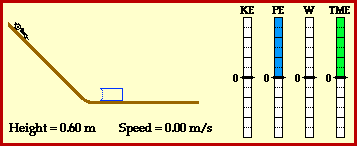## Stopping Distance of a Hot Wheels Car

Consider the motion of a Hot Wheels car beginning from rest at an elevated position. The Hot Wheels car rolls down a hill and begins its motion across a level surface. Along the level surface, the Hot Wheels car collides with a box and skids to a stop over a given distance. How could work and energy be utilized to analyze the motion of the Hot Wheels car? Would the total mechanical energy of the Hot Wheels car be altered in the process of rolling down the incline or in the process of skidding to a stop? Or would the total mechanical energy of the Hot Wheels car merely be conserved during the entire motion?

Of course the answers to these questions begin by determining whether or not external forces are doing work upon the car. If external forces do work upon the car, the total mechanical energy of the car is not conserved; the initial amount of mechanical energy is not the same as the final amount of mechanical energy. On the other hand, if external forces do not do work upon the car, then the total mechanical energy is conserved; that is, mechanical energy is merely transformed from the form of potential energy to the form of kinetic energy while the total amount of the two forms remains unchanged.

While the Hot Wheels car moves along the incline, external forces do not do work upon it. This assumes that dissipative forces such as air resistance have a negligible affect on the car's motion. This is a reasonable assumption for the low speeds of the car and its streamline characteristics. Since external forces do not do work on the car, the total mechanical energy of the car is conserved while moving along the incline. As the work-energy bar charts in the animation below depict, energy is transformed from potential energy (the stored energy of position) to kinetic energy (the energy of motion). The car gains speed as it loses height. The bar chart also depicts the fact that the total amount of mechanical energy is always the same; when the two forms are added together, the sum is unchanging.

When the Hot Wheels car collides with the box and skids to a stop, external forces do a significant amount of work upon the car. The force of friction acts in the direction opposite the car's motion and thus does negative work upon the car. This negative works contributes to a loss in mechanical energy of the car. In fact, if 0.40 Joules of mechanical energy are lost, then -0.40 Joules of work are done upon the car. As this work is done, the mechanical energy of the car (in the form of kinetic energy) is transformed into non-mechanical forms of energy such as sound and heat.

Analyze the animation and use the principles of work and energy to answer the given questions.Questions to Ponder:

1. Use energy conservation principles to determine the speed of a 0.050-kg Hot Wheels car that descends from a height of 0.60 meter to a height of 0.00 meter. Assume negligible air resistance.

2. Use energy conservation principles to determine the speed of a 0.050-kg Hot Wheels car that descends halfway down a 0.60-meter high hill (i.e., to a height of 0.30 meters). Assume negligible air resistance.

3. If the mass of the Hot Wheels car was twice as great (0.100 kg), then what would be the speed at the bottom of the 0.60-meter high hill?

4. If the 0.050-kg Hot Wheels car is brought to a rest over a distance of 0.40 meters, then what is the magnitude of the frictional force acting upon the car?

For more information on physical descriptions of motion, visit The Physics Classroom Tutorial. Detailed information is available there on the following topics:

Potential Energy

Kinetic Energy

Mechanical Energy

Internal vs. External Forces

Work

Work-Energy Theorem

Work-Energy Bar Charts